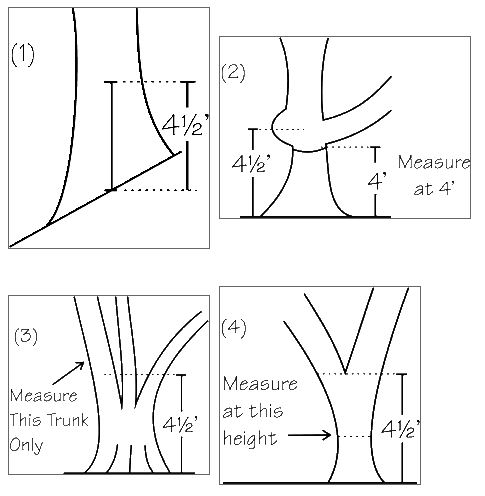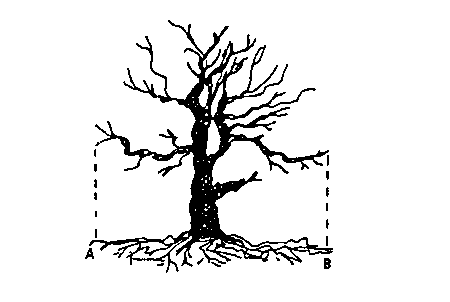# How to Measure a Champion Tree

Use the following methods to measure your tree.

## Circumference MeasurementUsing a flexible tape measure, measure the distance around the trunk of the tree to the nearest inch. This measurement should be taken at 4½ feet above ground level.

If the tree is on a slope (1), use the mid-point of the tree base to measure 4½ feet above ground level.

If there is a branch or growth on the trunk at 4½ feet (2), measure the circumference just below the obstruction and report the height at which the measurement was taken.

For multi-trunked trees that branch below 4½ feet (3), report the circumference of the largest trunk at 4½ feet.

If a multi-trunked tree flares out at 4½ feet (4), measure the smallest circumference below 4½ feet and report the height at which the measurement was taken.

## Height MeasurementTake a 12-inch ruler and hold it vertically at eye level, in an outstretched arm. Stand far enough away from the tree so that you can roughly see both the base and the top of the tree between the top and bottom of the ruler. Move forward or backward until the eye sights the base of the tree (A) across the 0-inch gradation (a) and the tip of the crown (B) across the 10-inch gradation (b). Then a sight is taken across the one inch (c) gradation and the corresponding point (C) is marked on the tree by a companion. Using a tape measure, measure the distance from the base of the tree to this point (AC) to the nearest foot and multiply by 10. This is the height of the tree (AB). If a height measuring instrument is available, its use is preferred. Be sure to report your method of measurement and have someone else verify your results.Place a marker under the outside edge of the crown that is farthest from the trunk (A) and another directly opposite it at the outer edge of the crown (B). Next, set a marker at the edge of the crown that is closest to the trunk (C) and another at the outer edge of the crown directly opposite it (D).Using a tape measure, measure both the distance from A to B and the distance from C to D to the nearest foot. Add the two measurements together and divide the sum by 2 to obtain the average crown spread.

## Point Value

The total point value, according to American Forests, is calculated as follows:

Total Points = Circumference in inches + Height in feet + ¼ of Average Crown Spread in feet